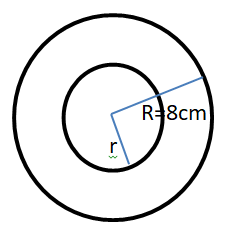Courses
Courses for Kids
Free study material
Offline Centres
MoreLast updated date: 22nd Nov 2023
Total views: 380.7k
Views today: 8.80k

# Volume of a hollow sphere is $\dfrac{{11352}}{7}{\text{ c}}{{\text{m}}^3}$ . If the outer radius is 8cm, find the inner radius of the sphere. (Take $\pi = \dfrac{{22}}{7}$ )Verified
380.7k+ views
Hint- If the hollow sphere has its outer radius as R and inner radius as small r then volume of the sphere is given as $V = \dfrac{4}{3}\pi ({R^3} - {r^3})$ . Using this formula we will find our solution.
Let $R$ and $r$ be the outer and inner radii of the hollow sphere respectively.
Let $V$ be the volume of the hollow sphere.Given that volume of the sphere is
$V = \dfrac{{11352}}{7}{\text{ c}}{{\text{m}}^3}$
$R = 8cm$
$\Rightarrow V = \dfrac{4}{3}\pi ({R^3} - {r^3}) \\ \Rightarrow \dfrac{{11352}}{7} = \dfrac{4}{3} \times \dfrac{{22}}{7}({8^3} - {r^3}) \\$
On simplifying above equation for the value of $r$ , we obtain
$\Rightarrow \dfrac{{11352 \times 3}}{{22 \times 4}} = {8^3} - {r^3} \\ \Rightarrow 387 = 512 - {r^3} \\ \Rightarrow {r^3} = 512 - 387 \\ \Rightarrow {r^3} = 125 \\ \Rightarrow r = 5cm \\$
Hence, the inner radius of the hollow sphere is, $r = 5cm$